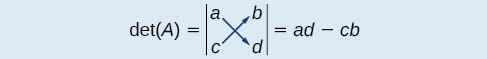## Evaluating the Determinant of a 2×2 Matrix

A determinant is a real number that can be very useful in mathematics because it has multiple applications, such as calculating area, volume, and other quantities. Here, we will use determinants to reveal whether a matrix is invertible by using the entries of a square matrix to determine whether there is a solution to the system of equations. Perhaps one of the more interesting applications, however, is their use in cryptography. Secure signals or messages are sometimes sent encoded in a matrix. The data can only be decrypted with an invertible matrix and the determinant. For our purposes, we focus on the determinant as an indication of the invertibility of the matrix. Calculating the determinant of a matrix involves following the specific patterns that are outlined in this section.

### A General Note: Find the Determinant of a 2 × 2 Matrix

The determinant of a $2\text{ }\times \text{ }2$ matrix, given

$A=\left[\begin{array}{cc}a& b\\ c& d\end{array}\right]$

is defined asFigure 1

Notice the change in notation. There are several ways to indicate the determinant, including $\mathrm{det}\left(A\right)$ and replacing the brackets in a matrix with straight lines, $|A|$.

### Example 1: Finding the Determinant of a 2 × 2 Matrix

Find the determinant of the given matrix.

$A=\left[\begin{array}{cc}5& 2\\ -6& 3\end{array}\right]$

### Solution

$\begin{array}{l}\mathrm{det}\left(A\right)=|\begin{array}{cc}5& 2\\ -6& 3\end{array}|\hfill \\ =5\left(3\right)-\left(-6\right)\left(2\right)\hfill \\ =27\hfill \end{array}$

## Using Cramer’s Rule to Solve a System of Two Equations in Two Variables

We will now introduce a final method for solving systems of equations that uses determinants. Known as Cramer’s Rule, this technique dates back to the middle of the 18th century and is named for its innovator, the Swiss mathematician Gabriel Cramer (1704–1752), who introduced it in 1750 in Introduction à l’Analyse des lignes Courbes algébriques. Cramer’s Rule is a viable and efficient method for finding solutions to systems with an arbitrary number of unknowns, provided that we have the same number of equations as unknowns.

Cramer’s Rule will give us the unique solution to a system of equations, if it exists. However, if the system has no solution or an infinite number of solutions, this will be indicated by a determinant of zero. To find out if the system is inconsistent or dependent, another method, such as elimination, will have to be used.

To understand Cramer’s Rule, let’s look closely at how we solve systems of linear equations using basic row operations. Consider a system of two equations in two variables.

$\begin{array}{c}{a}_{1}x+{b}_{1}y={c}_{1}\left(1\right)\\ {a}_{2}x+{b}_{2}y={c}_{2}\left(2\right)\end{array}$

We eliminate one variable using row operations and solve for the other. Say that we wish to solve for $x$. If equation (2) is multiplied by the opposite of the coefficient of $y$ in equation (1), equation (1) is multiplied by the coefficient of $y$ in equation (2), and we add the two equations, the variable $y$ will be eliminated.

$\begin{array}\text{ }b_{2}a_{1}x+b_{2}b_{1}y=b_{2}c_{1} \hfill& \text{Multiply }R_{1}\text{ by }b_{2} \\−b_{1}a_{2}x−b_{1}b_{2}y=−b_{1}c_{2} \hfill& \text{Multiply }R_{2}\text{ by }−b_{2} \\ \text{______________________} \\ b_{2}a_{1}x−b_{1}a_{2}x=−b_{2}c_{1}−b_{1}c_{2}\end{array}$

Now, solve for $x$.

$\begin{array}{l}{b}_{2}{a}_{1}x-{b}_{1}{a}_{2}x={b}_{2}{c}_{1}-{b}_{1}{c}_{2}\hfill \\ x\left({b}_{2}{a}_{1}-{b}_{1}{a}_{2}\right)={b}_{2}{c}_{1}-{b}_{1}{c}_{2}\hfill \\ \text{ }x=\frac{{b}_{2}{c}_{1}-{b}_{1}{c}_{2}}{{b}_{2}{a}_{1}-{b}_{1}{a}_{2}}=\frac{\left[\begin{array}{cc}{c}_{1}& {b}_{1}\\ {c}_{2}& {b}_{2}\end{array}\right]}{\left[\begin{array}{cc}{a}_{1}& {b}_{1}\\ {a}_{2}& {b}_{2}\end{array}\right]}\hfill \end{array}$

Similarly, to solve for $y$, we will eliminate $x$.

$\begin{array}\text{ }a_{2}a_{1}x+a_{2}b_{1}y=a_{2}c_{1} \hfill& \text{Multiply }R_{1}\text{ by }a_{2} \\−a_{1}a_{2}x−a_{1}b_{2}y=−a_{1}c_{2} \hfill& \text{Multiply }R_{2}\text{ by }−a_{1} \\ \text{______________________} \\ a_{2}b_{1}y−a_{1}b_{2}y=a_{2}c_{1}−a_{1}c_{2}\end{array}$

Solving for $y$ gives

$\begin{array}{l}{a}_{2}{b}_{1}y-{a}_{1}{b}_{2}y={a}_{2}{c}_{1}-{a}_{1}{c}_{2}\hfill \\ y\left({a}_{2}{b}_{1}-{a}_{1}{b}_{2}\right)={a}_{2}{c}_{1}-{a}_{1}{c}_{2}\hfill \\ \text{ }y=\frac{{a}_{2}{c}_{1}-{a}_{1}{c}_{2}}{{a}_{2}{b}_{1}-{a}_{1}{b}_{2}}=\frac{{a}_{1}{c}_{2}-{a}_{2}{c}_{1}}{{a}_{1}{b}_{2}-{a}_{2}{b}_{1}}=\frac{|\begin{array}{cc}{a}_{1}& {c}_{1}\\ {a}_{2}& {c}_{2}\end{array}|}{|\begin{array}{cc}{a}_{1}& {b}_{1}\\ {a}_{2}& {b}_{2}\end{array}|}\hfill \end{array}$

Notice that the denominator for both $x$ and $y$ is the determinant of the coefficient matrix.

We can use these formulas to solve for $x$ and $y$, but Cramer’s Rule also introduces new notation:

• $D:$ determinant of the coefficient matrix
• ${D}_{x}:$ determinant of the numerator in the solution of $x$
$x=\frac{{D}_{x}}{D}$
• ${D}_{y}:$ determinant of the numerator in the solution of $y$
$y=\frac{{D}_{y}}{D}$

The key to Cramer’s Rule is replacing the variable column of interest with the constant column and calculating the determinants. We can then express $x$ and $y$ as a quotient of two determinants.

### A General Note: Cramer’s Rule for 2×2 Systems

Cramer’s Rule is a method that uses determinants to solve systems of equations that have the same number of equations as variables.

Consider a system of two linear equations in two variables.

$\begin{array}{c}{a}_{1}x+{b}_{1}y={c}_{1}\\ {a}_{2}x+{b}_{2}y={c}_{2}\end{array}$

The solution using Cramer’s Rule is given as

$x=\frac{{D}_{x}}{D}=\frac{|\begin{array}{cc}{c}_{1}& {b}_{1}\\ {c}_{2}& {b}_{2}\end{array}|}{|\begin{array}{cc}{a}_{1}& {b}_{1}\\ {a}_{2}& {b}_{2}\end{array}|},D\ne 0;\text{ }\text{ }y=\frac{{D}_{y}}{D}=\frac{|\begin{array}{cc}{a}_{1}& {c}_{1}\\ {a}_{2}& {c}_{2}\end{array}|}{|\begin{array}{cc}{a}_{1}& {b}_{1}\\ {a}_{2}& {b}_{2}\end{array}|},D\ne 0$.

If we are solving for $x$, the $x$ column is replaced with the constant column. If we are solving for $y$, the $y$ column is replaced with the constant column.

### Example 2: Using Cramer’s Rule to Solve a 2 × 2 System

Solve the following $2\text{ }\times \text{ }2$ system using Cramer’s Rule.

$\begin{array}{c}12x+3y=15\\ \text{ }2x - 3y=13\end{array}$

### Solution

Solve for $x$.

$x=\frac{{D}_{x}}{D}=\frac{|\begin{array}{rr}\hfill 15& \hfill 3\\ \hfill 13& \hfill -3\end{array}|}{|\begin{array}{rr}\hfill 12& \hfill 3\\ \hfill 2& \hfill -3\end{array}|}=\frac{-45 - 39}{-36 - 6}=\frac{-84}{-42}=2$

Solve for $y$.

$y=\frac{{D}_{y}}{D}=\frac{|\begin{array}{rr}\hfill 12& \hfill 15\\ \hfill 2& \hfill 13\end{array}|}{|\begin{array}{rr}\hfill 12& \hfill 3\\ \hfill 2& \hfill -3\end{array}|}=\frac{156 - 30}{-36 - 6}=-\frac{126}{42}=-3$

The solution is $\left(2,-3\right)$.

### Try It 1

Use Cramer’s Rule to solve the 2 × 2 system of equations.

$\begin{array}{l}\text{ }x+2y=-11\hfill \\ -2x+y=-13\hfill \end{array}$